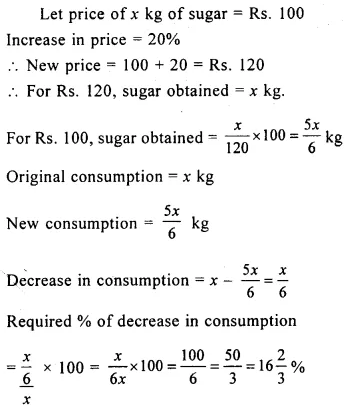# Selina Concise Mathematics Class 8 ICSE Solutions Chapter 7 Percent and Percentage

## Selina Concise Mathematics Class 8 ICSE Solutions Chapter 7 Percent and Percentage

Selina Publishers Concise Mathematics Class 8 ICSE Solutions Chapter 7 Percent and Percentage

### Percent and Percentage Exercise 7A – Selina Concise Mathematics Class 8 ICSE Solutions

Question 1.
Evaluate :
(i) 55% of 160 + 24% of 50 – 36% of 150
(ii) 9.3% of 500 – 4.8% of 250 – 2.5% of 240
Solution:Question 2.
(i) A number is increased from 125 to 150; find the percentage increase.
(ii) A number is decreased from 125 to 100; find the percentage decrease.
Solution: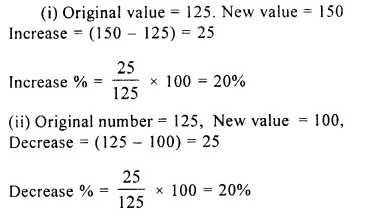Question 3.
Find :
(i) 45 is what percent of 54?
(ii) 2.7 is what percent of 18?
Solution:Question 4.
(i) 252 is 35% of a certain number, find the number.
(ii) If 14% of a number is 315; find the number.
Solution:Question 5.
Find the percentage change, when a number is changed from :
(i) 80 to 100
(ii) 100 to 80
(iii) 6.25 to 7.50
Solution: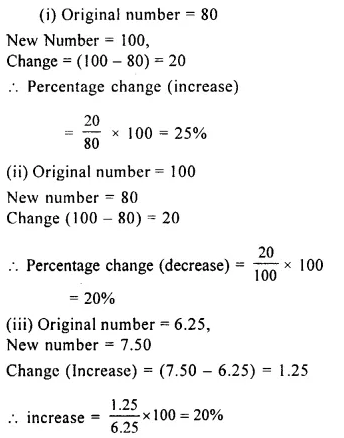Question 6.
An auctioneer charges 8% for selling a house. If a house is sold for Rs.2, 30, 500; find the charges of the auctioneer.
Solution: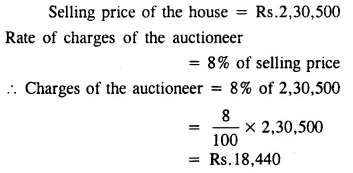Question 7.
Out of 800 oranges, 50 are rotten. Find the percentage of good oranges.
Solution: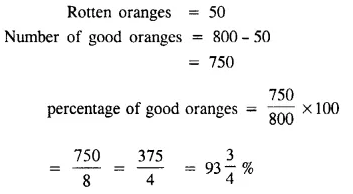Question 8.
A cistern contains 5 thousand litres of water. If 6% water is leaked. Find how many litres of water are left in the cistern.
Solution:Question 9.
A man spends 87% of his salary. If he saves Rs.325; find his salary.
Solution:Question 10.
(i) A number 3.625 is wrongly read as 3.265; find the percentage error.
(ii) A number 5.78 × 103 is wrongly written as 5.87 × 103; find the percentage error
Solution:Question 11.
In an election between two candidates, one candidate secured 58% of the votes polled and won the election by 18, 336 votes. Find the total number of votes polled and the votes secured by each candidate.
Solution:Question 12.
In an election between two candidates, one candidate secured 47% of votes polled and lost the election by 12, 366 votes. Find the total votes polled and die votes secured by the winning candidate.
Solution: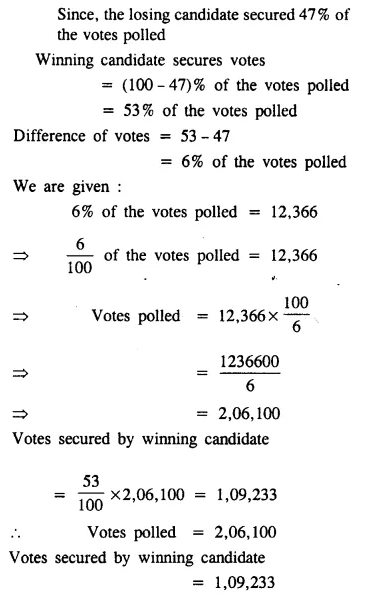Question 13.
The cost of a scooter depreciates every year by 15% of its value at the beginning of the year. If the present cost of the scooter is
₹ 8,000; find its cost:
(i) after one year
(ii) after 2 years
Solution: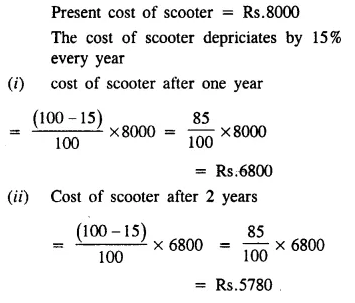Question 14.
In an examination, the pass mark is 40%. If a candidate gets 65 marks and fails by 3 marks; find the maximum marks.
Solution:Question 15.
In an examination, a candidate secured 125 marks and failed by 15 marks. If the pass percentage was 35 %; find the maximum marks.
Solution: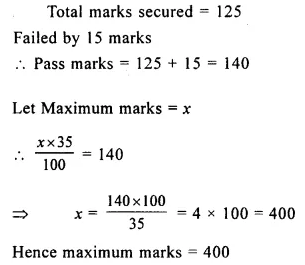Question 16.
In an objective type paper of 150 questions; John got 80% correct answers and Mohan got 64% correct answers.
(i) How many correct answers did each get?
Solution: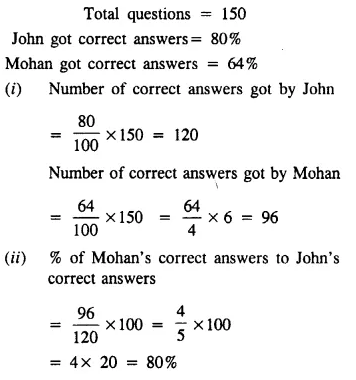Question 17.
The number 8,000 is first increased by 20% and then decreased by 20%. Find the resulting number.
Solution: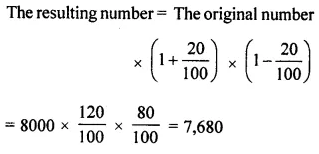Question 18.
The number 12,000 is first decreased by 25% and then increased by 25%. Find the resulting number.
Solution:Question 19.
The cost of an article is first increased by 20% and then decreased by 30%, find the percentage change in the cost of the article.
Solution:Question 20.
The cost of an article is first decreased by 25% and then further decreased by 40%. Find the percentage change in the cost of the article.
Solution:### Percent and Percentage Exercise 7B – Selina Concise Mathematics Class 8 ICSE Solutions

Question 1.
A man bought a certain number of oranges ; out of which 13 percent were found rotten. He gave 75% of the remaining in charity and still has 522 oranges left. Find how many had he bought?
Solution: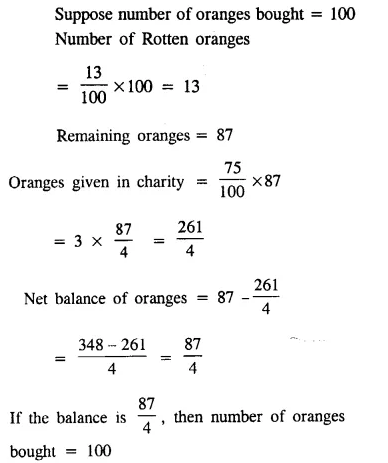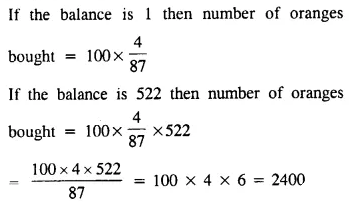Question 2.
5% pupil in a town died due to some diseases and 3% of the remaining left the town. If 2, 76, 450 pupil are still in the town; find the original number of pupil in the town.
Solution: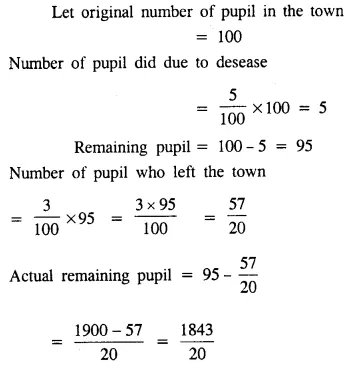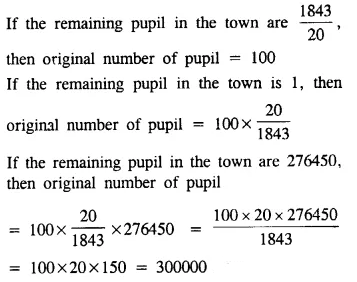Question 3.
In a combined test in English and Physics; 36% candidates failed in English; 28% failed in Physics and 12% in both; find:
(i) the percentage of passed candidates
(ii) the total number of candidates appeared, if 208 candidates have failed.
Solution: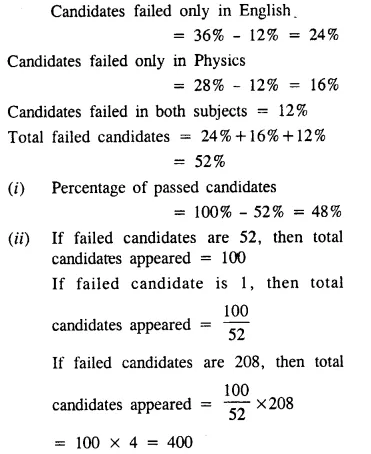Question 4.
In a combined test in Maths and Chemistry; 84% candidates passsed in Maths; 76% in Chemistry and 8% failed in both. Find :
(i) the percentage of failed candidates ;
(ii) if 340 candidates passed in the test ; then how many appeared?
Solution: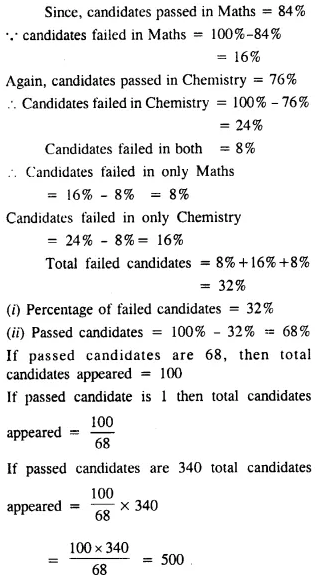Question 5.
A’s income is 25% more than B’s. Find, B’s income is how much percent less than A’s.
Solution: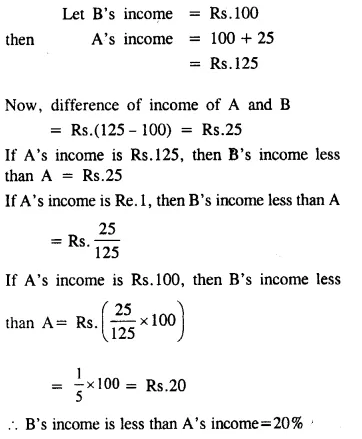Question 6.
Mona is 20% younger than Neetu. How much percent is Neetu older than Mona?
Solution:Question 7.
If the price of sugar is increased by 25% today; by what percent should it be decreased tomorrow to bring the price back to the original?
Solution: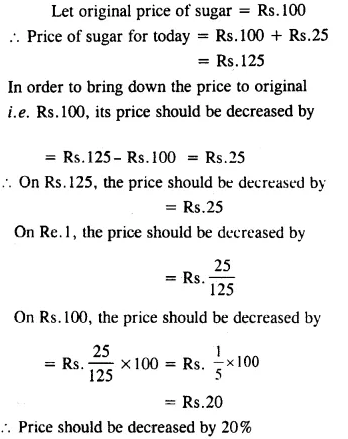Question 8.
A number increased by 15% becomes 391. Find the number.
Solution:Question 9.
A number decreased by 23 % becomes 539. Find the number.
Solution: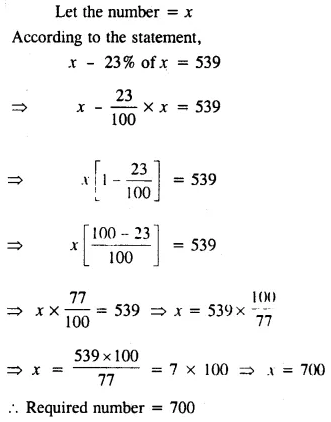Question 10.
Two numbers are respectively 20 percent and 50 percent more than a third number. What percent is the second of the first?
Solution:Question 11.
Two numbers are respectively 20 percent and 50 percent of a third number. What percent is the second of the first?
Solution:Question 12.
Two numbers are respectively 30 percent and 40 percent less than a third number. What percent is the second of the first?
Solution: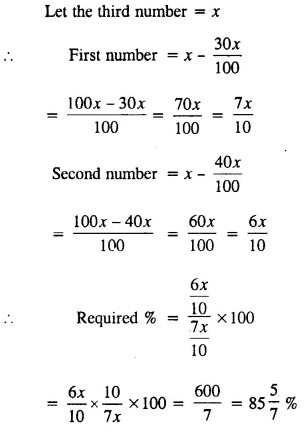### Percent and Percentage Exercise 7C – Selina Concise Mathematics Class 8 ICSE Solutions

Question 1.
A bag contains 8 red balls, 11 blue balls and 6 green balls. Find the percentage of blue balls in the bag.
Solution: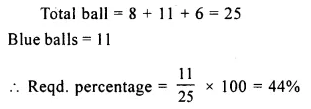Question 2.
Mohan gets Rs. 1, 350 from Geeta and Rs. 650 from Rohit. Out of the total money that Mohan gets from Geeta and Rohit. what percent does he get from Rohit?
Solution: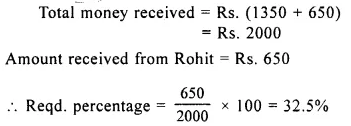Question 3.
The monthly income of a man is Rs. 16, 000. 15 percent of it is paid as income-tax and 75% of the remainder is spent on rent, food, clothing, etc. How much money is still left with the man?
Solution:Question 4.
A number is first increased by 20% and the resulting number is then decreased by 10%. Find the overall change in the number as percent.
Solution:Question 5.
A number is increased by 10% and the resulting number is again increased by 20%. What is the overall percentage increase in the number?
Solution: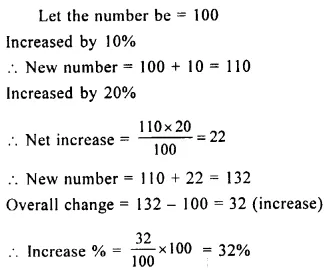Question 6.
During 2003, the production of a factory decreased by 25%. But, during 2004, it (production) increased by 40% of what it was at the beginning of2004. Calculate the resulting change (increase or decrease) in production during these two years.
Solution:Question 7.
Last year, oranges were available at Rs. 24 per dozen ; but this year, they are available at Rs. 50 per score. Find the percentage change in the price of oranges.
Solution: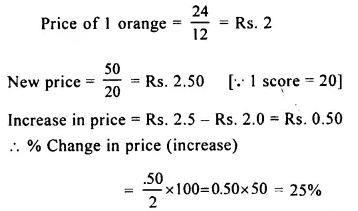Question 8.
In an examination, Kavita scored 120 out of 150 in Maths, 136 out of 200 in English and 108 out of 150 in Science. Find her percentage score in each subject and also on the whole (aggregate).
Solution: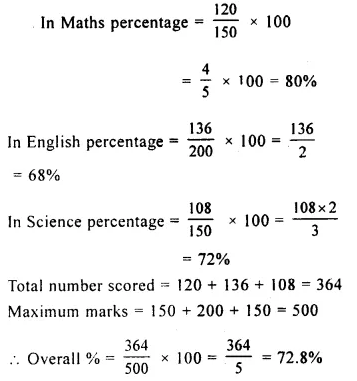Question 9.
A is 25% older than B. By what percent is B younger than A ?
Solution: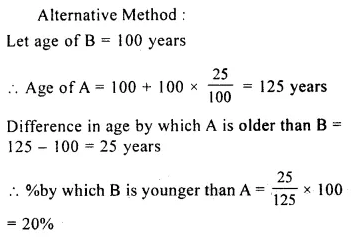Question 10.
(i) Increase 180 by 25%.
(ii) Decrease 140 by 18%.
Solution: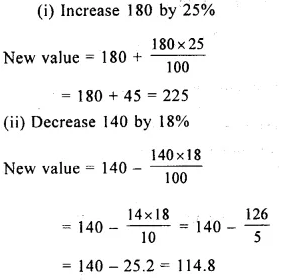Question 11.
In an election, three candidates contested and secured 29200, 58800 and 72000 votes. Find the percentage of votes scored by winning candidate.
Solution:Question 12.
(i) A number when increased by 23% becomes 861 ; find the number.
(ii) A number when decreased by 16% becomes 798 ; find the number.
Solution:Question 13.
The price of sugar is increased by 20%. By what percent must the consumption of sugar be decreased so that the expenditure on sugar may remain the same?
Solution: